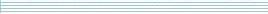### Some relationships between formal power series and nonnegative matrices

Speaker
Prof. T. Laffey
Duration
44:29
Date
17 October 2011
Abstract
Let σ = (λ_1,...,λ_n) be a list of complex numbers which we aim to realize constructively as the spectrum of a nonnegative matrix. Most constructions available in the literature rely on building matrices related to companion matrices from the polynomial f(x) = (x-λ_1)...(x-λ_n). Kim, Ormes and Roush (JAMS 2000) showed how certain formal power series related to f(x), which have all coefficients, other than the leading one, negative, can be used in finding constructions over the semiring of polynomials with nonnegative coefficients, while, in joint work, Šmigoc and this author (ELA 17 (2008) 333-342, LAMA 58 (2010), 1053-1059) have used polynomials having all their non-leading coefficients negative, to find realizations when σ has not more than two entries with positive real parts. Beginning with the observation that if λ_1,...,λ_n are all positive, then the Taylor expansion of the nth root of F(t) = (1-λ_1t)...(1-λ_nt) about t=0 has all its non-leading coefficients negative, we present a number of results on the negativity of the coefficients of power series and their applications to nonnegative matrices.
You should see the video here…
High quality
Fullscreen
Volume